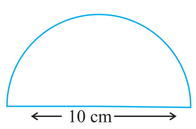Q

# Find the perimeter of the adjoining figure, which is a semicircle including its diameter.

Q. 7.     Find the perimeter of the adjoining figure, which is a semicircle including its diameter.Views

It is given that the diameter of semi-circle is 10 cm.

We know that

Circumference of semi circle is

Circumference of semi-circle with diameter 10 cm  including diameter is

Therefore, Circumference of semi-circle with diameter 10 cm  including diameter is 25.7 cm

Exams
Articles
Questions